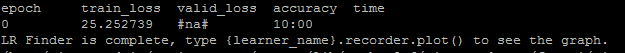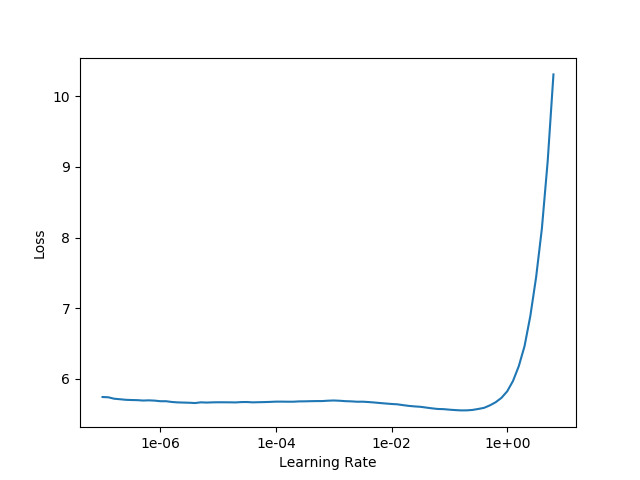# Lr_find: valid_loss is nan, curve flat

Hello,

I ran learn.lr_find(start_lr=1e-8, end_lr=1e2, num_it=100) to find the optimal learning rate for fine-tuning a language model. However, the validation loss is nan and the curve I get when running learn.recorder.plot() is very flat. Does someone know any explanation for either of the two issues?

Thanks for any suggestion!1 Like

Hi !

• The validation loss is NaN because we don’t track its value. Here we want to find an optimal lr value for the training of our model and thus we check its training loss
• Your curve is really flat because it reaches really high values at the end, which squishes your curve. There are two ways to solve that. (1) you change the value of `end_lr` to a lower one and (2) when using the function `learn.recorder.plot()`, there is an argument `skip_end` which will plot your curve by skipping some values at the end, if you increase this number, you should see a better curve.

Hope that helps !

6 Likes

Thanks so much for the clarification and hints! The graph looks a lot better now with the new settings!

Thanks for the calcification, I was also wondering the same thing. But this could be more clear in the documentation, `#na#` looks like something is wrong.

1 Like

Hi,

When I run fit - what is this number highlighted in yellow ? And when the epoch is finished - it runs a small run as well. What is the significance of these numbers.
The sceenshot if from lesson 6 - Rossman

Regards,
Abhinav

A little old, but thought I’d answer since I just came across this thread. The highlighted number in yellow is the total items / batch size = # of batches to run through. Its a more granular look than epochs, and less granular than total number of items processed.

1 Like